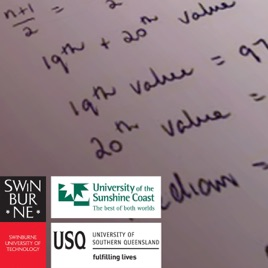24 Folgen

StatsCasts are narrated screen video recordings of explanations of statistical concepts. They are produced by Swinburne University of Technology, the University of the Sunshine Coast, and the University of Southern Queensland. They are part of an ongoing collaborative research project to develop high quality resources and investigate the effectiveness of StatsCasts to support statistics learning. They are targeted at prerequisite to first year level, in a range of subjects such as: Engineering, Sciences, Health Sciences and Business.# StatsCasts Swinburne University of Technology

• Bildung

StatsCasts are narrated screen video recordings of explanations of statistical concepts. They are produced by Swinburne University of Technology, the University of the Sunshine Coast, and the University of Southern Queensland. They are part of an ongoing collaborative research project to develop high quality resources and investigate the effectiveness of StatsCasts to support statistics learning. They are targeted at prerequisite to first year level, in a range of subjects such as: Engineering, Sciences, Health Sciences and Business.

• video
The Chi-square test (StatsCasts)

## The Chi-square test (StatsCasts)

In this video we look at an example of conducting a Chi-square test. We set up the null and alternative hypotheses, determine the significance level, calculate expected frequencies and the Chi-square statistic and use statistical tables to determine the critical value of the Chi-square statistic. We then use this information to make a decision about H0 and to write a conclusion.

• 7 Min.
• video
Calculation of probabilities in a Poisson distribution, using tables - Part 2 (StatsCasts)

## Calculation of probabilities in a Poisson distribution, using tables - Part 2 (StatsCasts)

In this video we look at how to use statistical tables to calculate probabilities in a Poisson distribution. This includes an example of using the table for the probability density function to determine the probability the random variable is equal to particular value in a case where the average number of events per interval needs to be adjusted to match the units specified in the question and an example of using the table for the cumulative distribution function to determine the probability the random variable takes a value between two specified numbers.

• 6 Min.
• video
Calculation of probabilities in a Poisson distribution, using tables - Part 1 (StatsCasts)

## Calculation of probabilities in a Poisson distribution, using tables - Part 1 (StatsCasts)

In this video we look at how to use statistical tables to calculate probabilities in a Poisson distribution. This includes an example of using the table for the probability density function to determine the probability the random variable is equal to a particular value and an example of using the table for the cumulative distribution function to determine the probability the random variable is less than a certain value and an example determining the probability it is greater than or equal to a certain value.

• 6 Min.
• video
Calculation of probabilities in a Binomial distribution, using tables (StatsCasts)

## Calculation of probabilities in a Binomial distribution, using tables (StatsCasts)

In this video we look at how to use statistical tables to calculate probabilities in a Binomial distribution. This includes an example of using the table for the probability density function to determine the probability the random variable takes a particular value and an example of using the table for the cumulative distribution function to determine the probability the random variable is less than or equal to a certain value and an example determining the probability it is greater than or equal to a certain value.

• 7 Min.
• video
Deciding if a distribution is Binomial or Poisson (StatsCasts)

## Deciding if a distribution is Binomial or Poisson (StatsCasts)

In this video we look at how to decide for a given scenario (worded problem) if the distribution described is a Binomial distribution or Poisson distribution and whether its probability distribution function or its cumulative distribution function is required to calculate a specified probability.

• 7 Min.
• video
Background to test statistic and p value in z test (StatsCasts)

## Background to test statistic and p value in z test (StatsCasts)

An example of the step by step process used to conduct a z test for the mean, with emphasis on the interpretation of the test statistic (z) and the p-value.

• 8 Min.